Home » Degrees vs Mils

# Degrees vs Mils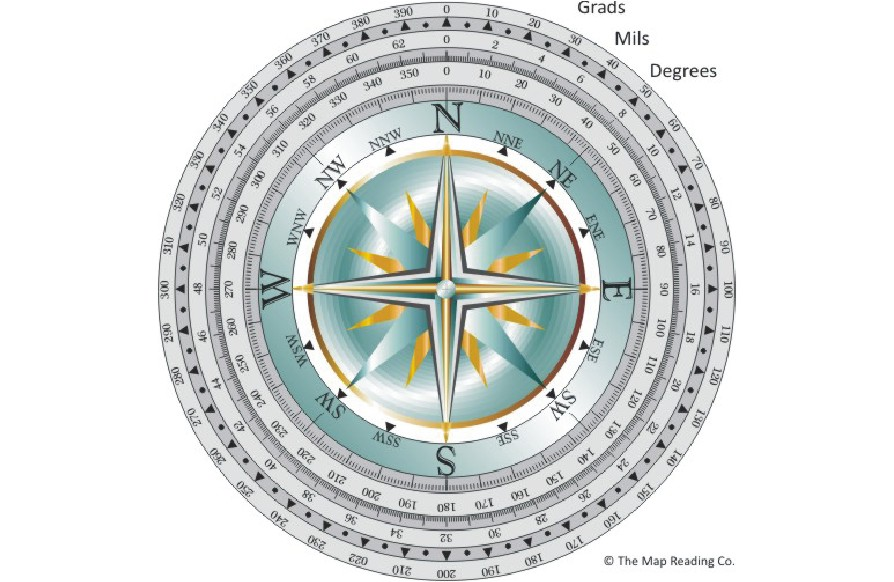See here
YouTube will open in a new tab.

A brief look at the differences between compasses with Degrees and Mils.

Most compasses have one thing in common; they will give us a number which signifies a direction.

If someone else understands the number they will also understand the direction, without us having to point, explain or draw a line on a map.

There are many different numbering systems used to describe direction from a given point. For example the lake is 15 degrees from the edge of the forest. So in this case the given point is edge of the forest and if we were to follow a direction of 15 degrees we would eventually come to the lake.

There are also many different systems for dividing a circle into units.  For example Grads / Gradians which are mostly used by land surveyors. The reason they are useful for surveying, but not navigating, is that in the Grad system each right angle is divided in to 100 units (Centigrads), so a complete circle is 400. As land surveyors tend to use geometric shapes, as opposed to North, South, etc, using Grads makes the maths involved much simpler.

The most common numbers used when navigating with a map and compass are Degrees and Mils.

Degrees.

When you were at school you were told (as a fact) that a circle has 360 degrees. You weren’t told why 360 was a magic number, why not 456 or 987, or better still 100. Even though 360 is just an arbitrary number, it really doesn’t matter, as long as everyone uses the same, system 360 is as good a number as any other.

The reason we divide a circle into 360 units is down to the ancient Greeks, Babylonians and others who used the stars to work out when to plant their crops, make sacrifices to the gods, etc. They knew that certain constellations of stars would be in a slightly different place each night and these constellations would go all the way around the sky each year and return to the same point once a year. As they used a calendar which had 12 months, each with 30 days, a full circle must be 12 X 30.  This is why a circle has 360 units – or degrees: from the old French word degré, meaning a step.

Of course we now know that the Earth orbits around the sun every 365.256 days, so 360 is not too bad, and it’s much simpler to use in school maths lessons.

On a compass North is shown as 0 degrees, East is 90, South is 180 and West is 270.

However, in most cases of land navigation (at sea it’s different), the direction is simply given as a number. For example let’s walk on a bearing of 130 degrees for ten minutes, or from the peak the wall junction is 400m at 140 degrees.

Mils.

Mils (short for Mil-Radians) are, almost exclusively, used by the Military as they are ideal for shooting targets, directing artillery, etc.

I say almost exclusively as some people still believe they are ideal for walking and navigating with a map and compass – they are not.

An obvious drawback is that most people, when out walking and navigating, use Degrees and not Mils. So if you have taken a bearing in Mils and want to pass this on to someone else you are going to need a calculator to convert from one system to the other.

One of the fundamental differences between Degrees and Mils is that a circle is divided into 360 degrees or 6283.185 mils (normally rounded up to 6,400)

From our (map reading and navigation) view we can’t say that the Mil system must be more accurate as each unit (of a circle) is smaller, as this makes no sense in the real world.

It is simply not possible, using a hand-held compass, to take a bearing with an accuracy of better than 1 or 2 degrees. Then if you add in calculation errors when adjusting for magnetic declination plus the normal, lack of, walking accuracy when following a compass bearing, you can see why all competent navigators use a compass with Degrees rather than Mils.

Mils continued.

The vast majority of people using Mils don’t understand what they are and this makes no difference what-so-ever.

As long as you can use Mils (or Degrees for the matter) to navigate competently that’s fine.

In maths Radians and Mils are described as angles.

But from a navigation viewpoint there is a fundamental difference between Degrees and Mils and it is this: On a compass Degrees are angles and Mils are distances.

Both can be used to describe a direction.

Now this may seem like a slightly strange statement as how can you use a distance to define a direction?

To answer this question it’s necessary to remember our school maths lessons.

• A Circumference – the distance around the circle
• A Radius – the distance from the center to the Circumference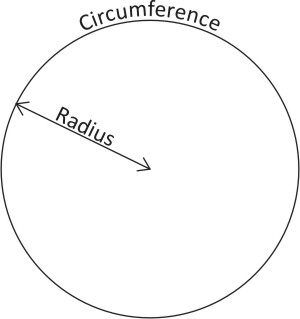In maths a Radian is the size of an angle that, when drawn from the center of a circle, touches the circumference at two points and the length of the arc between the points is the same as the radius.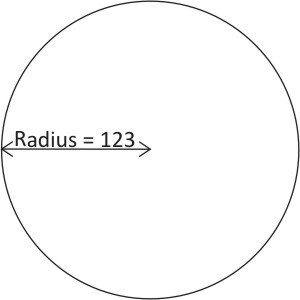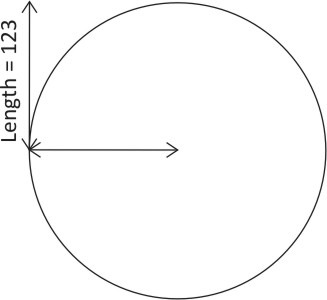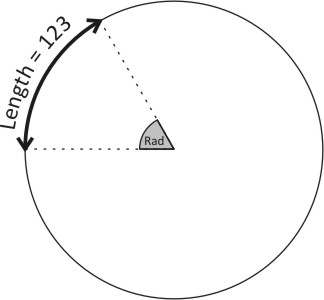But on a compass a Radian is not an angle, it is the length around part of the Circumference (known as an arc) which is equal to the Radius.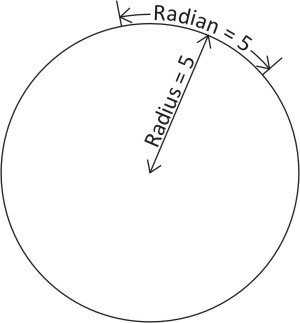So for example if you have a circle with a Radius of 5 (cm, feet, miles, etc.) the Radian is also 5.

A Radian can be divided in to 1,000 units called a Mil-Radians.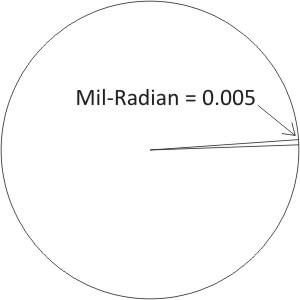Note that in the graphic the size of a Mil-Radian has been extremely enlarged so you can see it.

Military use of Mils.

Now it may not be apparent from simply looking at the Radius and Circumference of a circle, but THIS is why the military use Mils and not Degrees.

Don’t forget that maths goes both ways.

If you know the size of either a circle’s radius or circumference you can calculate the other.

It’s the same with using Mils.

If you know the either the size of something or its distance you can calculate the other.

• Let’s say you are looking through your scope and see a person (most people are approx. 2m tall) and they appear to be 2 mils from top to bottom using the dots marked on the lens.
• In this case each Mil-Radian is 1m – as they are 2m tall and covering 2 mils in your scope.
• So in this case a Radian is 1000m – as a Mil-Radian is 1,000th of a Radian.
• Don’t forget that a Radian equals the radius of a circle and you can imagine the person (in your scope) is on the Circumference.
• So, as they are 2 mils tall, they are 2km away.
• Size (cm) ÷ mils x 10 = Range (m)

Using the same principle you can adjust artillery fire which is missing its target and do lots of other “stuff” which the military does.

Civilian use of Mils

It may help to think about in this way:

• Imagine you are standing at the center of a circular running track.
• You see someone set off around the track and stop sometime later.
• Using Degrees you can say they started at some angle (relative to North) and they ended at another angle.
• Using Mils you would say they had moved a certain number of Mils around the track – from where they where to where they are. Each Mil is 1: 6,400th of the circumference of the track.
• As you can tell from the last statement, what really matters is how big the circular track is. If the radius (the distance from the center point to the circumference) of the running track is small they will have run less distance than if the radius were larger.
• They may have started at a point which your compass tells you is 2,345 Mils (0 being North) and finished at 3,456 Mils.
• So they have covered a distance of 1,111 Mils.  Don’t forget that equals 1,111 X 1:6,400th of the circumference.  The distance they travelled is only dependent on the size of the circle.
• Remember that on a compass Mils are distances not angles.
• You can always imagine yourself at the center of a circle with features on the circumference e.g. a lake, a peak, a crossroads, etc.
• And (importantly) you can be at the center of many circles at the same time, so each feature’s direction can be described in Mils.
• Remember that North is 0.
• So to use Mils to define a direction all you need to do is to is say that something/someone is at X number of Mils.  e.g. The lake is at 345 Mils.  If someone else understands that this means the Lake is 345 X 1:6,400th (clockwise) of a circular path from North, or has a similar compass, they will know which direction you are are talking about.

Note:  There are 6283 Mils in a circle, but on most compasses this is rounded up to 6,400